/

### Function Description

Returns the LU decomposition (without index and permutation sign) of a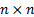matrix matrix. The LU decomposition is amatrix from which we can construct lower and upper diagonal matrices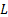and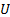such that if the input matrix is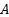then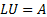. As per Press et al. (2007) the algorithm returns permuted lower and upper triangular matrices, with the permutations separately returned using MnMatLUIndexAndPerm.

Using the LU decomposition (together with the LU index and permutation sign) speeds up solution of matrix equations etc.

Contents | Prev | Next